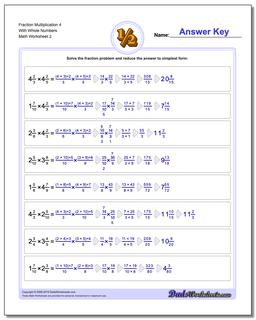# Math Worksheets: Fraction Multiplication: Fraction Multiplication: Fraction Multiplication 4 With Whole Numbers (Second Worksheet)## Fraction Multiplication 4 With Whole Numbers (Second Worksheet)

PropertyValue
DescriptionFraction Multiplication 4 With Whole Numbers: Basic fraction multiplication with whole parts, no cross-cancelling (Second Worksheet)
Resource TypeWorksheet